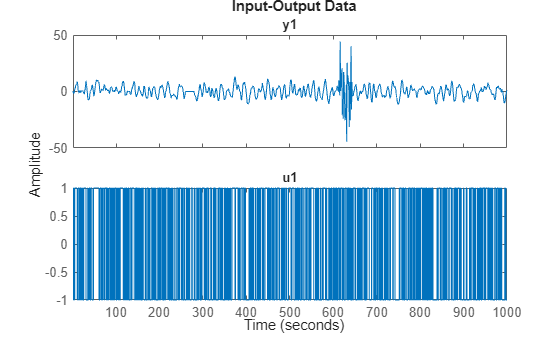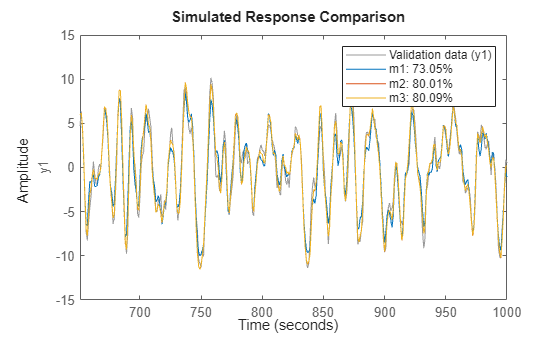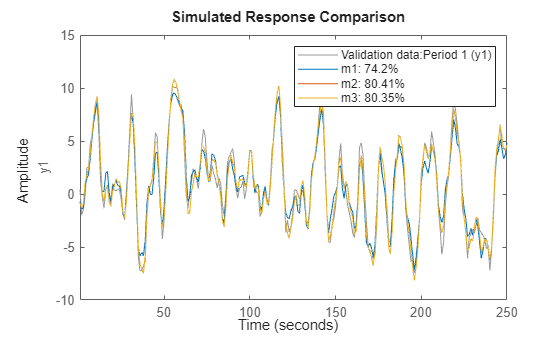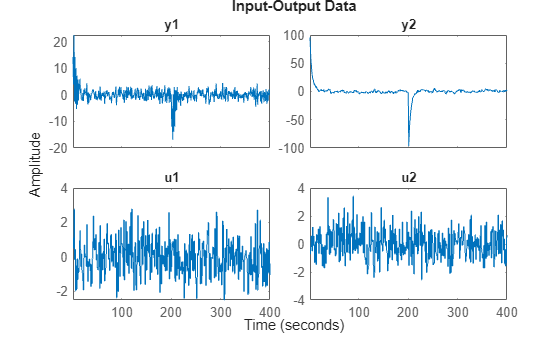Dealing with Multi-Experiment Data and Merging Models

This example shows how to deal with multiple experiments and merging models when working with System Identification Toolbox™ for estimating and refining models.

Introduction

The analysis and estimation functions in System Identification Toolbox let you work with multiple batches of data. Essentially, if you have performed multiple experiments and recorded several input-output datasets, you can group them up into a single IDDATA object and use them with any estimation routine.

In some cases, you may want to "split up" your (single) measurement dataset to remove portions where the data quality is not good. For example, portion of data may be unusable due to external disturbance or a sensor failure. In those cases, each good portion of data may be separated out and then combined into a single multi-experiment IDDATA object.

For example, let us look at the dataset iddemo8.mat:

The name of the data object is dat, and let us view it.

dat
dat =

Time domain data set with 1000 samples.
Sample time: 1 seconds

Outputs      Unit (if specified)
y1

Inputs       Unit (if specified)
u1

plot(dat)We see that there are some problems with the output around sample 250-280 and around samples 600 to 650. These might have been sensor failures.

Therefore split the data into three separate experiments and put then into a multi-experiment data object:

d1 = dat(1:250);
d2 = dat(281:600);
d3 = dat(651:1000);
d = merge(d1,d2,d3) % merge lets you create multi-exp IDDATA object
d =
Time domain data set containing 3 experiments.

Experiment   Samples      Sample Time
Exp1         250            1
Exp2         320            1
Exp3         350            1

Outputs      Unit (if specified)
y1

Inputs       Unit (if specified)
u1

The different experiments can be given other names, for example:

d.exp = {'Period 1';'Day 2';'Phase 3'}
d =
Time domain data set containing 3 experiments.

Experiment     Samples      Sample Time
Period 1       250            1
Day 2          320            1
Phase 3        350            1

Outputs        Unit (if specified)
y1

Inputs         Unit (if specified)
u1

To examine it, use plot, as in plot(d).

Performing Estimation Using Multi-Experiment Data

As mentioned before, all model estimation routines accept multi-experiment data and take into account that they are recorded at different periods. Let us use the two first experiments for estimation and the third one for validation:

de = getexp(d,[1,2]);      % subselection is done using  the command GETEXP
dv = getexp(d,'Phase 3');  % using numbers or names.
m1 = arx(de,[2 2 1]);
m2 = n4sid(de,2);
m3 = armax(de,[2 2 2 1]);
compare(dv,m1,m2,m3)The compare command also accepts multiple experiments. Use the right click menu to pick the experiment to use, one at a time.

compare(d,m1,m2,m3)Also, spa, etfe, resid, predict, sim operate in the same way for multi-experiment data, as they do for single experiment data.

Merging Models After Estimation

There is another way to deal with separate data sets: a model can be computed for each set, and then the models can be merged:

m4 = armax(getexp(de,1),[2 2 2 1]);
m5 = armax(getexp(de,2),[2 2 2 1]);
m6 = merge(m4,m5); % m4 and m5 are merged into m6

This is conceptually the same as computing m from the merged set de, but it is not numerically the same. Working on de assumes that the signal-to-noise ratios are (about) the same in the different experiments, while merging separate models makes independent estimates of the noise levels. If the conditions are about the same for the different experiments, it is more efficient to estimate directly on the multi-experiment data.

We can check the models m3 and m6 that are both ARMAX models obtained on the same data in two different ways:

[m3.a;m6.a]
ans = 2×3

1.0000   -1.5034    0.7008
1.0000   -1.5022    0.7000

[m3.b;m6.b]
ans = 2×3

0    1.0023    0.5029
0    1.0035    0.5028

[m3.c;m6.c]
ans = 2×3

1.0000   -0.9744    0.1578
1.0000   -0.9751    0.1584

compare(dv,m3,m6)Case Study: Concatenating Vs. Merging Independent Datasets

We now turn to another situation. Let us consider two data sets generated by the system m0. The system is given by:

m0
m0 =
Discrete-time identified state-space model:
x(t+Ts) = A x(t) + B u(t) + K e(t)
y(t) = C x(t) + D u(t) + e(t)

A =
x1        x2        x3
x1    0.5296    -0.476    0.1238
x2    -0.476  -0.09743    0.1354
x3    0.1238    0.1354   -0.8233

B =
u1        u2
x1    -1.146  -0.03763
x2     1.191    0.3273
x3         0         0

C =
x1       x2       x3
y1  -0.1867  -0.5883  -0.1364
y2   0.7258        0   0.1139

D =
u1     u2
y1  1.067      0
y2      0      0

K =
y1  y2
x1   0   0
x2   0   0
x3   0   0

Sample time: 1 seconds

Parameterization:
STRUCTURED form (some fixed coefficients in  A, B, C).
Feedthrough: on some input channels
Disturbance component: none
Number of free coefficients: 23
Use "idssdata", "getpvec", "getcov" for parameters and their uncertainties.

Status:
Created by direct construction or transformation. Not estimated.

The data sets that have been collected are z1 and z2, obtained from m0 with different inputs, noise and initial conditions. These datasets are obtained from iddemo8.mat that was loaded earlier.

pause off

First data set:

plot(z1) %generates a separate plot for each I/O pair if pause is on; showing only the last one hereThe second set:

plot(z2) %generates a separate plot for each I/O pair if pause is on; showing only the last one hereIf we just concatenate the data we obtained:

zzl = [z1;z2]
zzl =

Time domain data set with 400 samples.
Sample time: 1 seconds

Outputs      Unit (if specified)
y1
y2

Inputs       Unit (if specified)
u1
u2

plot(zzl)pause on

A discrete-time state-space model can be obtained by using ssest:

ml = ssest(zzl,3,'Ts',1, 'Feedthrough', [true, false]);

Compare the bode response for models m0 and ml:

clf
bode(m0,ml)
legend('show')This is not a very good model, as observed from the four Bode plots above.

Now, instead treat the two data sets as different experiments:

zzm = merge(z1,z2)
zzm =
Time domain data set containing 2 experiments.

Experiment   Samples      Sample Time
Exp1         200            1
Exp2         200            1

Outputs      Unit (if specified)
y1
y2

Inputs       Unit (if specified)
u1
u2

% The model for this data can be estimated as before (watching progress this time)
mm = ssest(zzm,3,'Ts',1,'Feedthrough',[true, false], ssestOptions('Display', 'on'));

Let us compare the Bode plots of the true system (blue)

the model from concatenated data (green) and the model from the

merged data set (red):

clf
bode(m0,'b',ml,'g',mm,'r')
legend('show')The merged data give a better model, as observed from the plot above.

Conclusions

In this example we analyzed how to use multiple data sets together for estimation of one model. This technique is useful when you have multiple datasets from independent experiment runs or when you segment data into multiple sets to remove bad segments. Multiple experiments can be packaged into a single IDDATA object, which is then usable for all estimation and analysis requirements. This technique works for both time and frequency domain iddata.

It is also possible to merge models after estimation. This technique can be used to "average out" independently estimated models. If the noise characteristics on multiple datasets are different, merging models after estimation works better than merging the datasets themselves before estimation.

System Identification Toolbox DocumentationGet trial now